## Selina Concise Mathematics Class 9 ICSE Solutions Graphical Solution (Solution of Simultaneous Linear Equations, Graphically)

APlusTopper.com provides step by step solutions for Selina Concise Mathematics Class 9 ICSE Solutions Chapter 27 Graphical Solution (Solution of Simultaneous Linear Equations, Graphically). You can download the Selina Concise Mathematics ICSE Solutions for Class 9 with Free PDF download option. Selina Publishers Concise Mathematics for Class 9 ICSE Solutions all questions are solved and explained by expert mathematic teachers as per ICSE board guidelines.

Selina ICSE Solutions for Class 9 Maths Chapter 27 Graphical Solution (Solution of Simultaneous Linear Equations, Graphically)

Exercise 27(A)

Solution 1:
(i)
The graph x= 5 in the following figure is a straight line AB which is parallel to y axis at a distance of 5 units from it.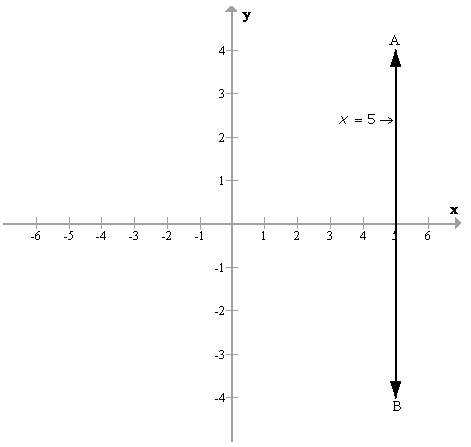(ii)
x+5=0
x = -5
The graph x= -5 in the following figure is a straight line AB which is parallel to y axis at a distance of 5 units from it in the negative x direction.(iii)
The graph y = 7 in the following figure is a straight line AB which is parallel to x axis at a distance of 7 units from it.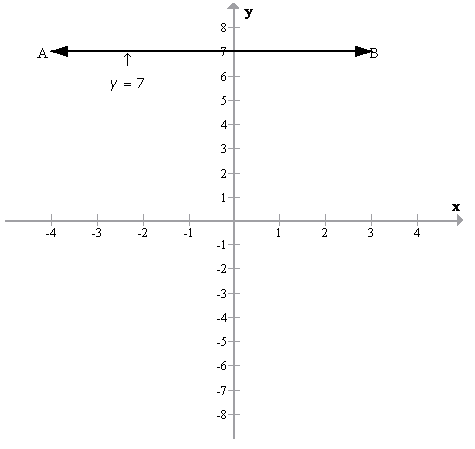(iv)
y + 7 = 0
y = -7
The graph y = -7 in the following figure is a straight line AB which is parallel to x axis at a distance of 7 units from it in the negative y direction.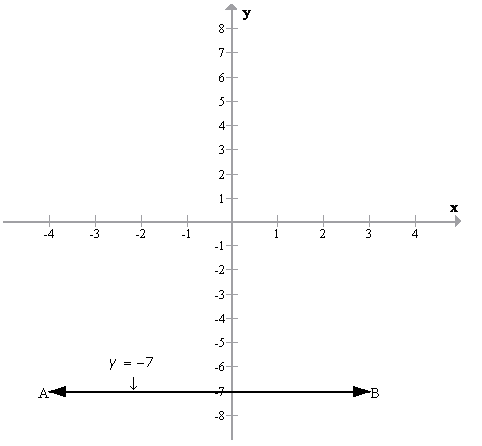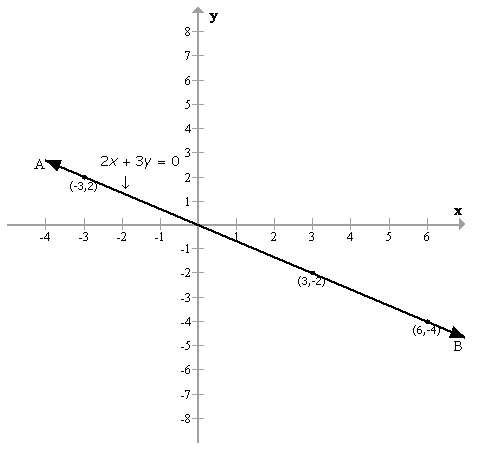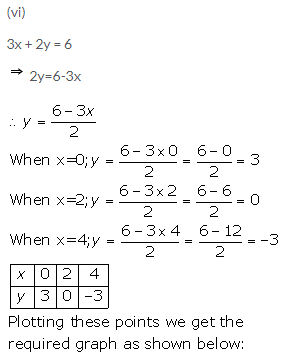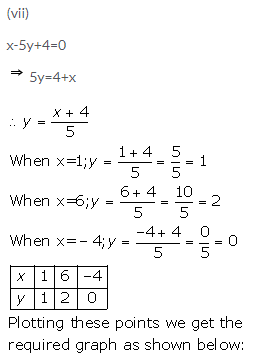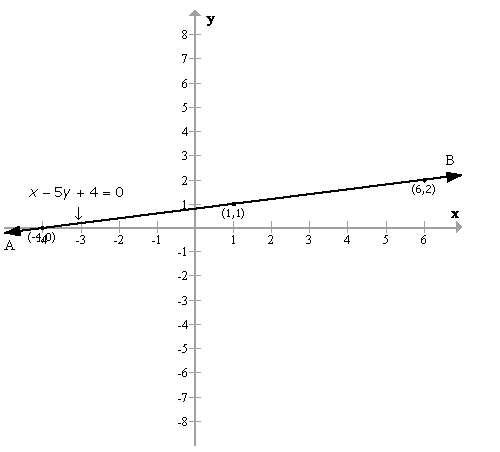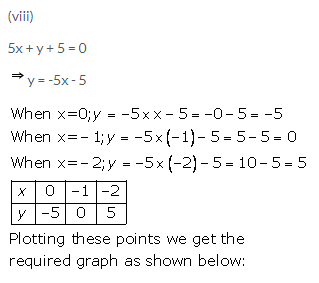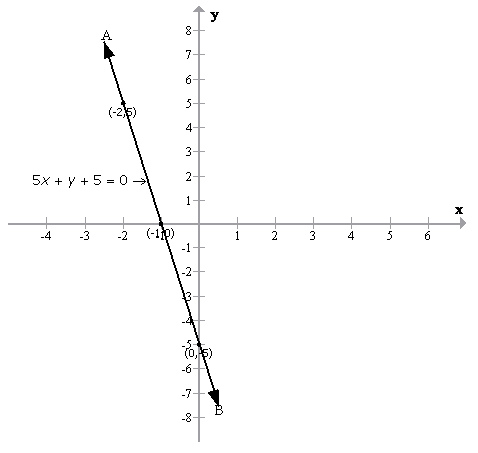Solution 2: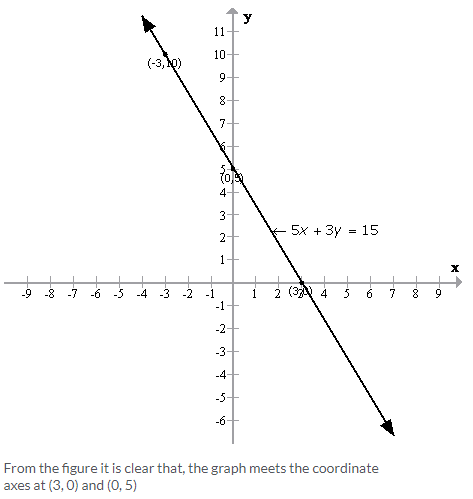From the figure it is clear that, the graph meets the coordinate axes at (-9, 0) and (0, 6)

Solution 3:Solution 4:Solution 5:Solution 6: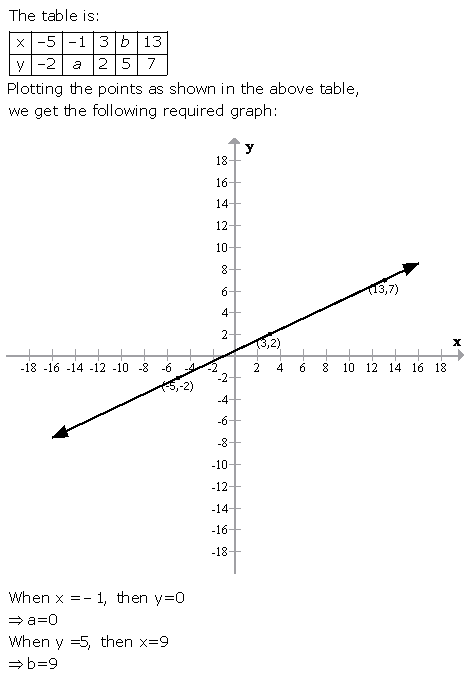Solution 7: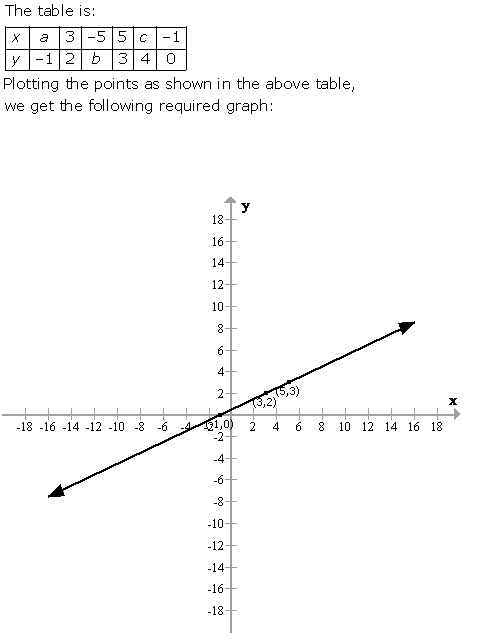Solution 8: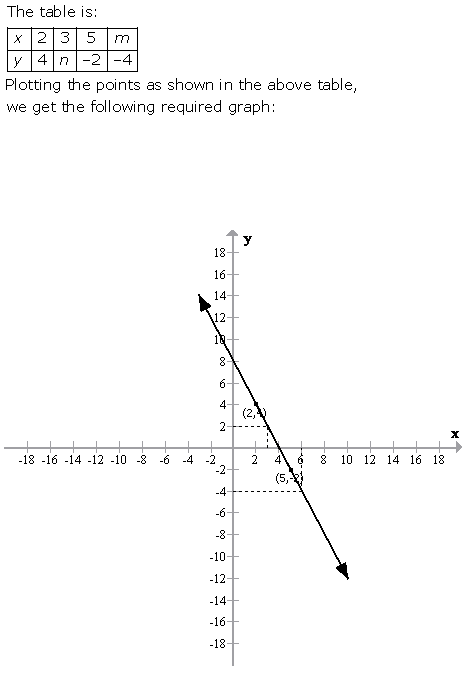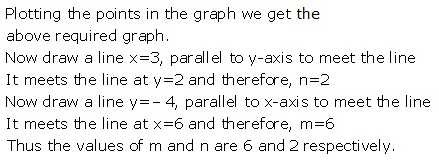Solution 9: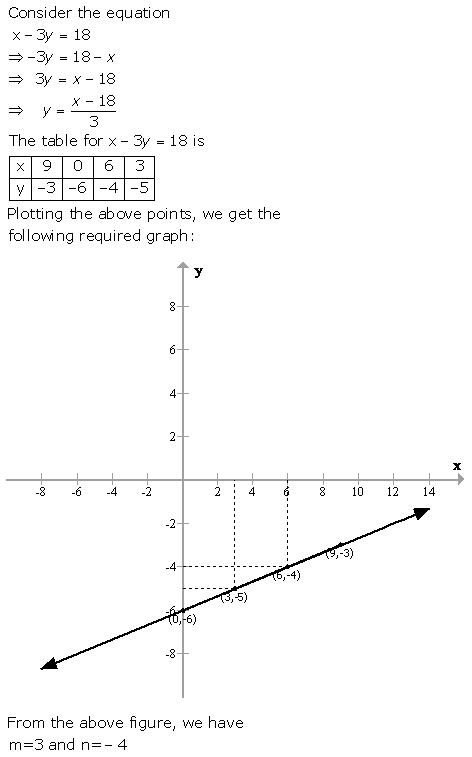Solution 10: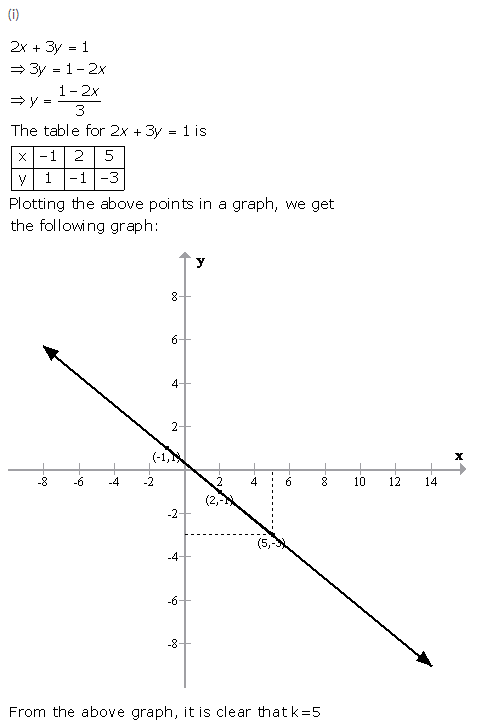Exercise 27(B)

Solution 1: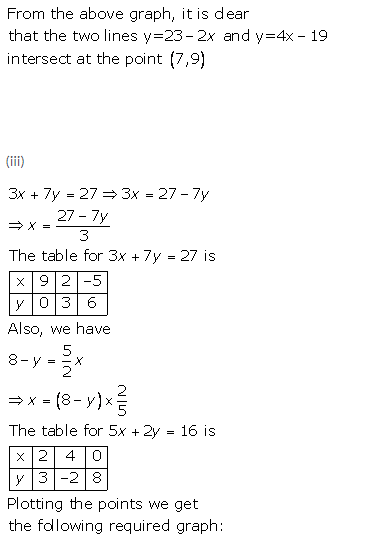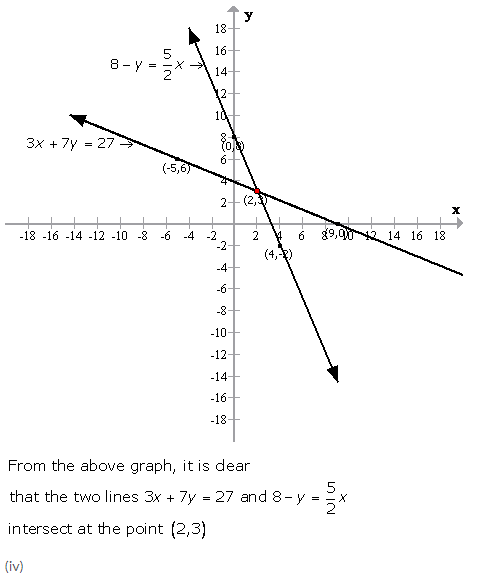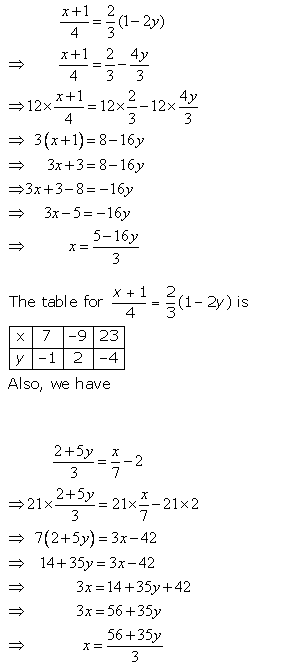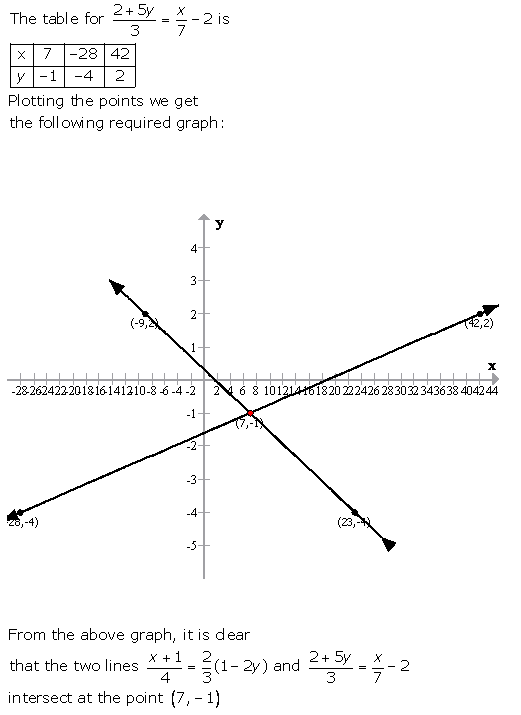Solution 2: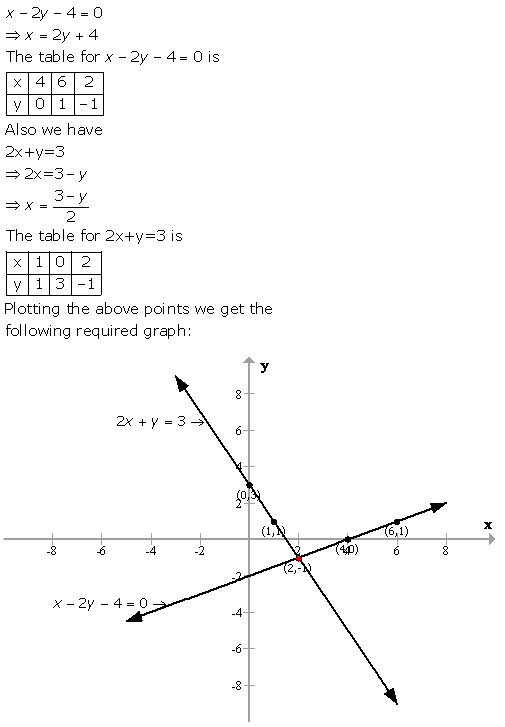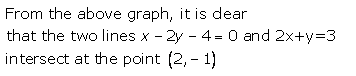Solution 3: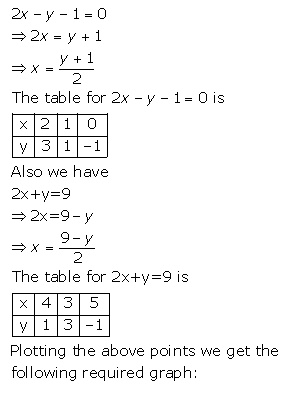Solution 4:Solution 5: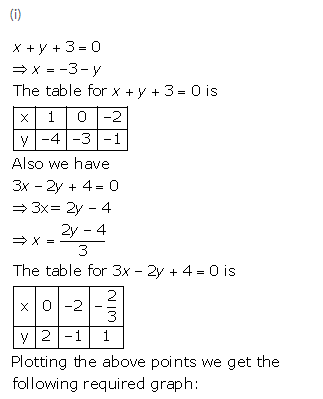Solution 6: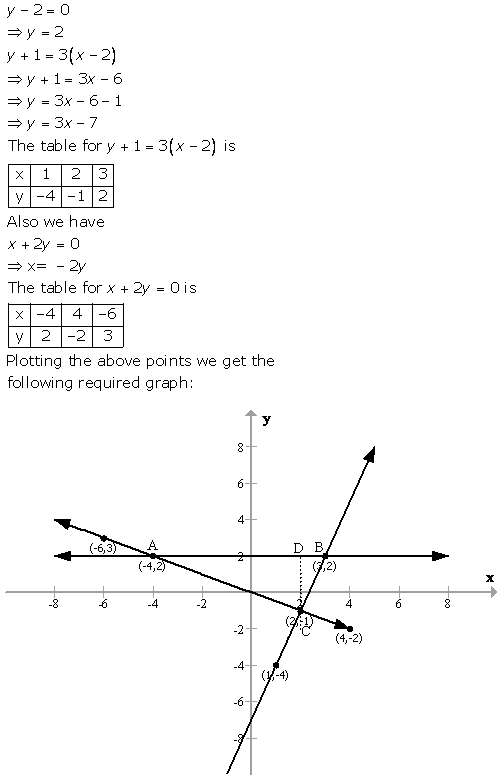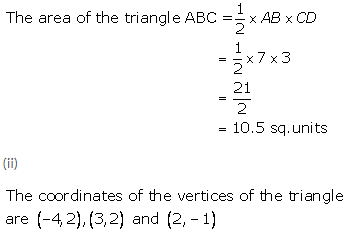Solution 7: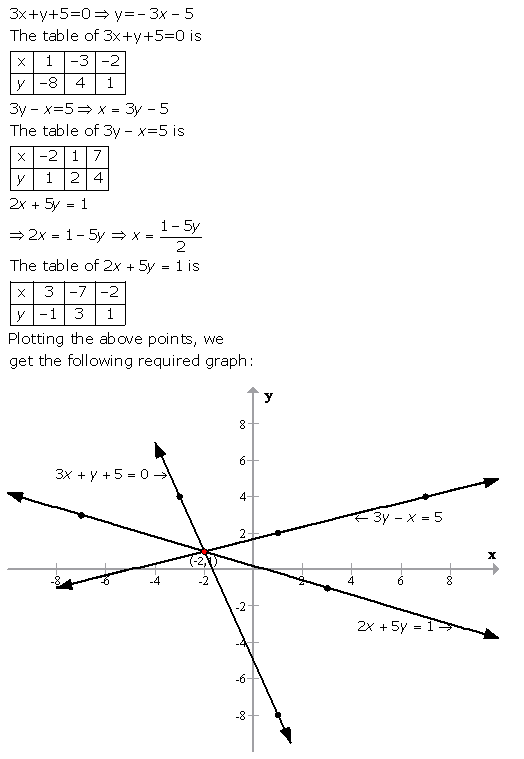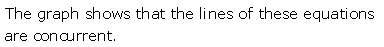Solution 8:Solution 9: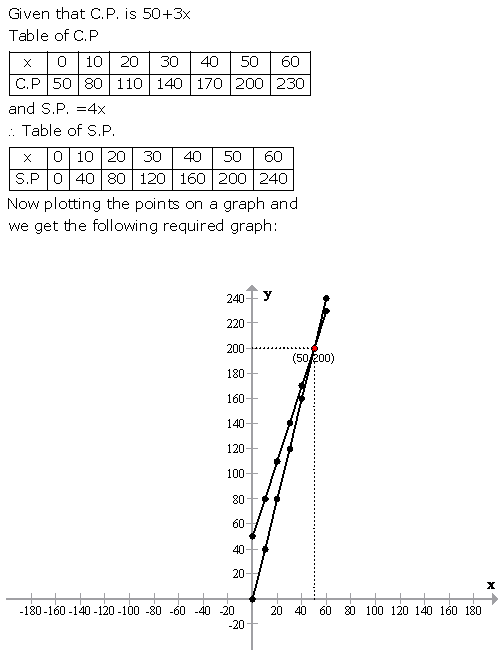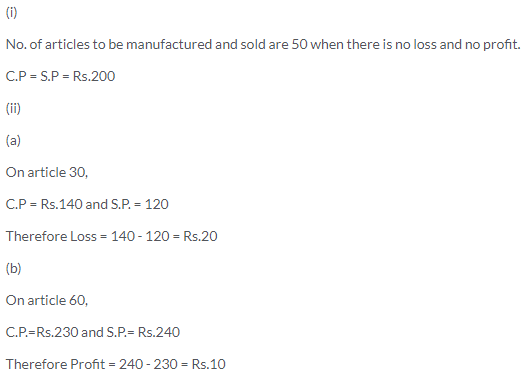Solution 10: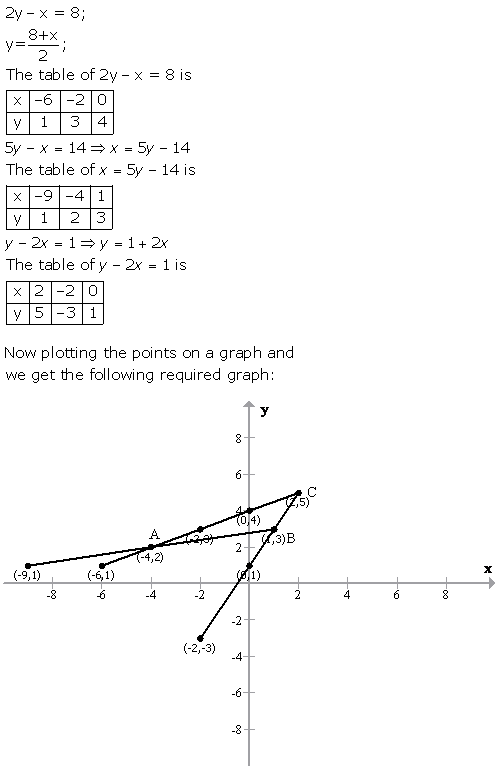Solution 11: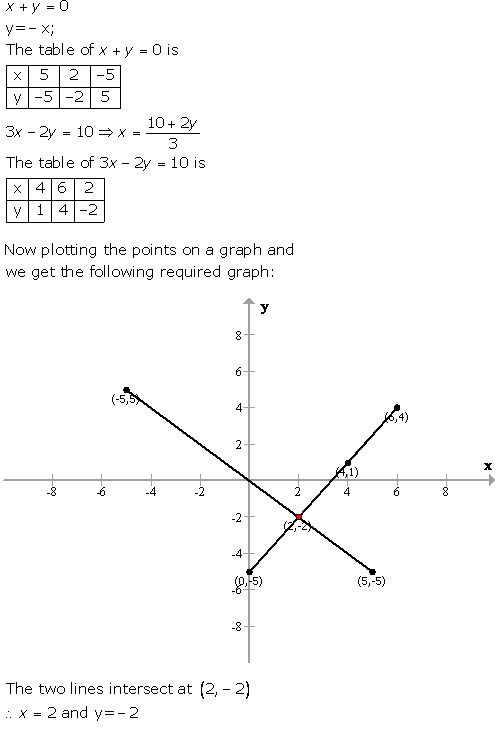Solution 12:Solution 13:Solution 14:More Resources for Selina Concise Class 9 ICSE Solutions